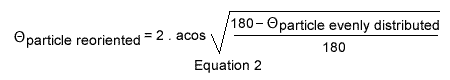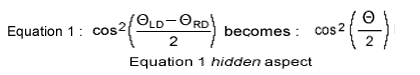## Hidden Variable

BEWARE, at time of this writing, the following language and equation is denied by most professional physicists; yet this gravimotion entanglement interpretation while concurring with science's mathematics is only challenging science's psychological interpretation of it.
Should mathematics not be your bag, simply ignore Equation 2 and jump to the paragraph printed in green color below.
Equation 2 below, while concurring with Quantum probabilities Equation 1, brings to light the individual behavior of each particle, as predicted by EPR yet banished by Bell's theorem.
Provided the pairs are evenly distributed when emitted, those tilted between 10 and 1350 represent three quarters of those tilted between 10 and 1800. The remaining 45 pairs between 1350 and 1800 make the remaining quarter. And due to symetry an identical distribution applies to the other 1810 to 3600 half of the spectrum.
In order to match the physical measures and Quantum Mechanics statistics when the detectors are set 1200 apart, it suffices to come up with an equation that modifies the pairs tilted between 00 and 1350 to angles tilted between 00 and 1200. Equation 2 is just doing that:When the detectors are oriented 1200 apart as in Figures 2 and 3, the particles evenly tilted from 00 to 1350 when emitted will be, according to Equation 2, deflected and measured between 00 and 1200 by both Detectors; these explicit deflections precisely coincide with the physical measures and Quantum mechanics statistics.
This explicit interpretation is written in green because it is in agreement with both experiments and Quantum Mechanics probabilities.

In this interpretation the 2 particles of any pair are acting in concert with Quantum probabilities, not because one side is instructing the other to comply instantly over distance, but because at the very time the measures take place, each and all electron tilts are individually and precisely deflected in the amount provided by Equation 2.

## Quantum Mechanics Equation 1 Hidden Aspect / Detector Effective Behavior

Gravimotion's Cyber Experiment shows that both left and right detectors are each behaving independently of each other in accordance to universal rules; and that occurs no matter the reference chosen by us human beings for our human interpretation; as such any reference other than the trajectory, as introduced in preceding Human Logic page, may be chosen.
Let us choose the ΘRD right Detector angular orientation for reference; ΘRD being now null:Equation 3, a derivative of Equation 1 hidden aspect shows that detectors' orientations just as electrons' tilts can be re-oriented in order to describe the entanglement phenomenon.Equation 3 modifies θDetectorNominalOrientation = 1200 into θDetectorEffectiveOrientation = 1350 and θDetectorNominalOrientation = 00 remains unchanged at θDetectorEffectiveOrientation = 00.
In these conditions one quarter identical measures instead of one third identical measures will occur, provided the emitted electrons are evenly distributed over the full 3600 circumference.

## Tacit entanglement

The two detectors MIRROR IMAGES of each other, because SET 120 DEGREES APART, are behaving in concert as if they were physically connected. Note that this tacit behavior interpretation turns out to be complying with Quantum Mechanics non-locality.
Yet the pairs' two particles COMPLEMENTARY state is also acting tacitly on each side of the emitter over distance just as if the 2 particles were connected through a physical rod playing the role of instantaneous communication betwen the two.
These just mentioned 2 tacits behaviors are now individually acting in concert with each other, on each side of the pairs' emitter, no matter the distance. This distant tacit collaboration is now justifying EPR's hidden variable.

When the detectors are set 120 degrees apart, Quantum Mechanics stipulates that only an infinite number of pairs emitted will provide the 25% 75% distribution. The Cyber Experiment proposed here reveals that 3 essential factors are actually at play:
1. The number of pairs must be a whole of 8.
2. The particles angular orientation must be evenly distributed over the entire 360 degrees spectrum; as such the number of pairs to be emitted can be 8, or 16, or 24, or 32 up to an infinite number the later only being considered in Quantum Mechanics theory and experiments.
Should one choose to emit 24 pairs, the pairs should be seperated 15 degrees from each other; such details, even though essential, are totally ignored in Quantum Mechanics.
3. And as mentioned above the pairs' complementary aspect is of the essence.
The computer simulation labeled Cyber Experiment reveals that this complementary factor may be interpreted either as the 2 particles of any pair being emitted at 180 degrees from each other or the two particles being emitted with identical orientation.
When complementary pairs are emitted 3 quarters flash same colors 1 quarter differing colors. When twins are emitted 1 quarter flash identical colors 3 quarters flash differing colors.
All pairs' configurations that are neither complementary nor twin fail to provide the 1 to 3 quarters distribution.
Yet all of above is not what creates the 25%, 75% distribution; such specific distribution is entirely dependent on the deflection of the particles. Or besides all of above, which is mandatory, the particles deflections (called diffration in science) are the cause behind the observed phenomenon.
In addition the Cyber Experiment proposed in this application disclosed another result usually not mentioned in science; the 25% 75% distribution is also occurring when the detectors' orientations differ by 60 degrees, 240 degrees and 300 degrees.